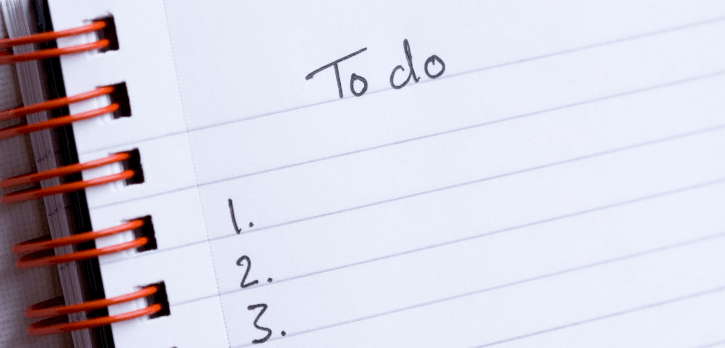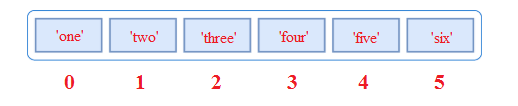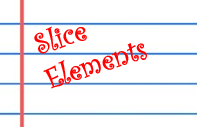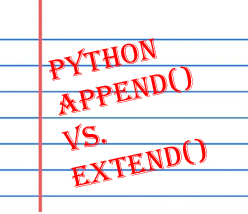# Python ListPython List is one of the most frequently used and very versatile datatype used in Python. In Python, lists are objects and it holds a number of other objects. Lists are very similar to arrays. It implements the sequence protocol, and also allows you to add and remove objects from the sequence. List literals are written within square brackets [ ] with the first element at index 0. There are many methods associated to them. Some of which are presented here below.

## Creating List

Creating a list is as simple as putting different comma-separated values in square brackets.

a_list = [1,2,3,4] b_list = ['a','b','c','d'] c_list = ['one','two','three','four','five,'six'] d_list = [1,2,'three','four']### Accessing List Values

The syntax for accessing the elements of a list is the same as the syntax for accessing the characters of a string. The expression inside the brackets specifies the index. Python indexes starts its lists at 0 rather than 1.

example
a_list = [1,2,3,4] num1 = a_list num2 = a_list print(num1) print(num2)
output
1 4
example
d_list = [1,2,'three','four'] num = d_list str = d_list print(num) print(str)
output
2 three

### List length

The function len returns the length of a list, which is equal to the number of its elements.

example
numbers = ['one','two','three','four','five'] list_len = len(numbers) print(list_len)
output
5

### Clear or Emptying List

list.clear() remove all items from the list.

example
numbers = ['one','two','three','four','five'] print(numbers) numbers.clear() print(numbers)
output
['one', 'two', 'three', 'four', 'five'] []

### Inserting and Removing Elements

append() - Appends adds its argument as a single element to the end of a list. The length of the list itself will increase by one.

example
numbers = ['one','two','three','four','five'] numbers.append('six') print(numbers)
output
['one', 'two', 'three', 'four', 'five', 'six']

### Appending a list inside a list

example
num1 =[1,2,3] num2 = [4,5,6] num1.append(num2) print(num1)
output
[1, 2, 3, [4, 5, 6]]

### List operations

Using the "+" operator concatenates lists.

example
num1 =[1,2,3] num2 = [4,5,6] num3 = num1 + num2 print(num3)
output
[1, 2, 3, 4, 5, 6]

using the * operator repeats a list a given number of times.

example
num1 =['hi'] num = num1 * 4 print(num)
output
['hi', 'hi', 'hi', 'hi']
example
num1 =[1,2,3,4] num = num1 * 2 print(num)
output
[1, 2, 3, 4, 1, 2, 3, 4]

### Inserting elements in Listexample
num1 =[1,2,4] num1.insert(2,3) #inserts an element into the third position print(num1)
output
[1, 2, 3, 4]
example
num1 =[1,2,3,4,6] num1.insert(-1,5) #inserts an element into the second from last position of the list (negative indices start from the end of the list) print(num1)
output
[1, 2, 3, 4, 5, 6]

### Remove lements from List

example
numbers = ['one','two','three','four','five'] numbers.remove('three') print(numbers)
output
['one', 'two', 'four', 'five']

### List Count

list.count(x) return the number of times x appears in the list.

example
str = ['h','e','l','l','o'] cnt = str.count('l') print(cnt)
output
2

### Slice Elements

Python slice extracts elements, based on a start and stop.

example
str = ['h','e','l','l','o'] lsc = str[1:4] print(lsc)
output
['e', 'l', 'l']str[1:4] - The 1 means to start at second element in the list (note that the slicing index starts at 0). The 4 means to end at the fifth element in the list, but not include it. The colon in the middle is how Python's lists recognize that we want to use slicing to get objects in the list.

example
str = ['h','e','l','l','o'] lsc = str[:3] # slice first three elements print(lsc)
output
['h', 'e', 'l']
example
str = ['h','e','l','l','o'] lsc = str[3:] # slice from 4th element, Python starts its lists at 0 rather than 1. print(lsc)
output
['l', 'o']

### List Reverse

The reverse() method in list reverse the elements of the list in place.

example
str = ['h','e','l','l','o'] str.reverse() print(str)
output
['o', 'l', 'l', 'e', 'h']

### List index()

The index() method returned the index of the first matching item.

example
str = ['h','e','l','l','o'] ind = str.index('l') # from start 'l' is in 2nd position print(ind)
output
2

If you want to specify a range of valid index, you can indicate the start and stop indices:

example
str = ['h','e','l','l','o'] ind = str.index('l',3) # start searching from 3rd position print(ind)
output
3

### Exist in List

We can test if an item exists in a list or not, using the keyword "in"

example
str = ['h','e','l','l','o'] print('e' in str)
output
True

### not in List

example
str = ['h','e','l','l','o'] print('e' not in str)
output
False

### Create new List with Dynamic Values

example
dList = [3 ** i for i in range(5)] print(dList)
output
[1, 3, 9, 27, 81]

### List sort

List sort() method that performs an in-place sorting

example
str = ['h','e','l','l','o'] str.sort() print(str)
output
['e', 'h', 'l', 'l', 'o']

### Reverse Sorting

example
str = ['h','e','l','l','o'] str.sort(reverse=True) print(str)
output
['o', 'l', 'l', 'h', 'e']

## List as StackA stack is a container of objects that are inserted and removed according to the last-in first-out (LIFO) principle. In the pushdown stacks only two operations are allowed: push the item into the stack, and pop the item out of the stack. Here to add an item to the top of the List stack, use append() (push) and to retrieve an item from the top of the stack, use pop() without an explicit index. example
stack = [1,2,3,4,5] print("Before push ", stack) stack.append(6) stack.append(7) print("After push ", stack) stack.pop() print("After pop ", stack) stack.pop() stack.pop() print("After pop ", stack)
output
Before push [1, 2, 3, 4, 5] After push [1, 2, 3, 4, 5, 6, 7] After pop [1, 2, 3, 4, 5, 6] After pop [1, 2, 3, 4]

## Lists as Queues

A queue is a container of objects that are inserted and removed according to the first-in first-out (FIFO) principle. In the queue only two operations are allowed enqueue and dequeue. Enqueue (append()) means to insert an item into the back of the queue, dequeue (pop(0)) means removing the front item. example
queue = [1,2,3] print("Before enqueue ", queue) queue.append(4) queue.append(5) print("After enqueue ", queue) queue.pop(0) print("After dequeue ", queue) queue.pop(0) queue.pop(0) print("After dequeue ", queue)
output
Before enqueue deque([1, 2, 3]) After enqueue deque([1, 2, 3, 4, 5]) After dequeue deque([2, 3, 4, 5]) After dequeue deque([4, 5])

### Iterating Through a List

Using a for loop we can iterate though each item in a list.

example
str = ['h','e','l','l','o'] for s in str: print(s)
output
h e l l o

In order to get every other item, starting with the first.

example
str = [1,2,3,4,5,6,7,8,9,10] print(str[::2])
output
[1, 3, 5, 7, 9]

Get every other item, starting with the second.

example
str = [1,2,3,4,5,6,7,8,9,10] print(str[1::2])
output
[2, 4, 6, 8, 10]

### Reverse items

example
str = [1,2,3,4,5,6,7,8,9,10] print(str[::-1])
output
[10, 9, 8, 7, 6, 5, 4, 3, 2, 1]

### zip() function

To loop over two or more sequences at the same time, the entries can be paired with the zip() function.

example
numbers = [1,2,3,4,5] alpla = ['a','b','c','d','e'] for num, alp in zip(numbers,alpla): print(num, alp)
output
1 a 2 b 3 c 4 d 5 e

## How to remove duplicates from a Python List

The common approach to get a unique collection of items is to use a dictionary. A Python dictionary is a mapping of unique keys to values. So, converting Python list to dictionary will automatically remove any duplicates because dictionaries cannot have duplicate keys . example
numList = [1,2,3,1,4,2,5,3] numList = list(dict.fromkeys(numList)) print(numList)
output
[1, 2, 3, 4, 5]

## Python list extend method()

The list extend() method extends the list by adding all items of a list (passed as an argument) to the end.
list.extend(anotherList)
example
list1 = ['a', 'b', 'c'] list1.extend(['d', 'e']) print (list1)
output
['a', 'b', 'c', 'd', 'e']

## Difference between list methods append() and extend()?Python append() method adds an element to a list, and the extend() method concatenates the first list with another list (or another iterable). When append() method adds its argument as a single element to the end of a list, the length of the list itself will increase by one. Whereas extend() method iterates over its argument adding each element to the list, extending the list. The length of the list will increase by however many elements were in the iterable argument. Python append() example
list1 = ['a', 'b', 'c'] list1.append(['d', 'e']) print (list1)
output
['a', 'b', 'c', ['d', 'e']]
Python extends() example
list1 = ['a', 'b', 'c'] list1.extend(['d', 'e']) print (list1)
output
['a', 'b', 'c', 'd', 'e']